# Graph Transformations

A LevelAQAEdexcelOCR

## Graph Transformations

You should have seen some graph transformations before, such as translations and reflections – recall that reflections in the $x$-axis flip $f(x)$ vertically and reflections in the $y$-axis flip $f(x)$ horizontally. Here, we will also look at stretches.

There are 4 main types of graph transformation that we will cover. Each transformation has the same effect on all functions.

Make sure you are happy with the following topics before continuing.

A LevelAQAEdexcelOCR

## Type 1: $y = f(x+k)$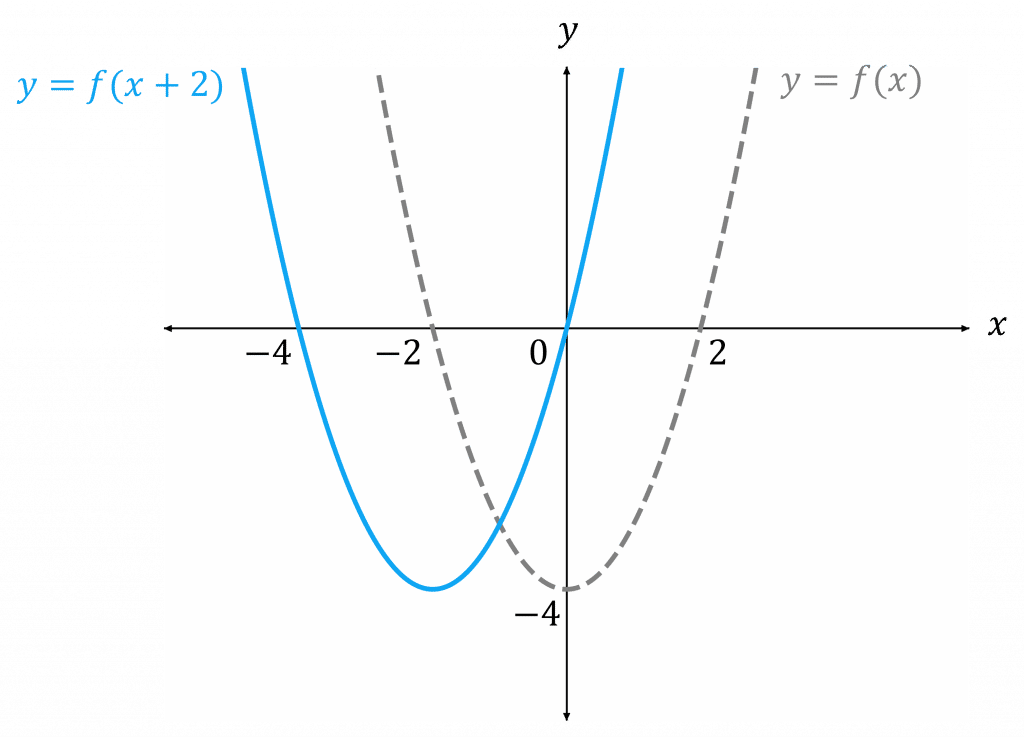For the transformation $y=f(x+k)$, for $k>0$:

• $f(x+k)$ is $f(x)$ moved $k$ to the left
• $f(x-k)$ is $f(x)$ moved $k$ to the right

In this example, we have $f(x) = x^2 - 4$ and $y=f(x+2)$

So, subtract $2$ from the $x$-coordinates of $f(x)$ to get $y=f(x+2)$

A LevelAQAEdexcelOCR

## Type 2: $y = f(x)+k$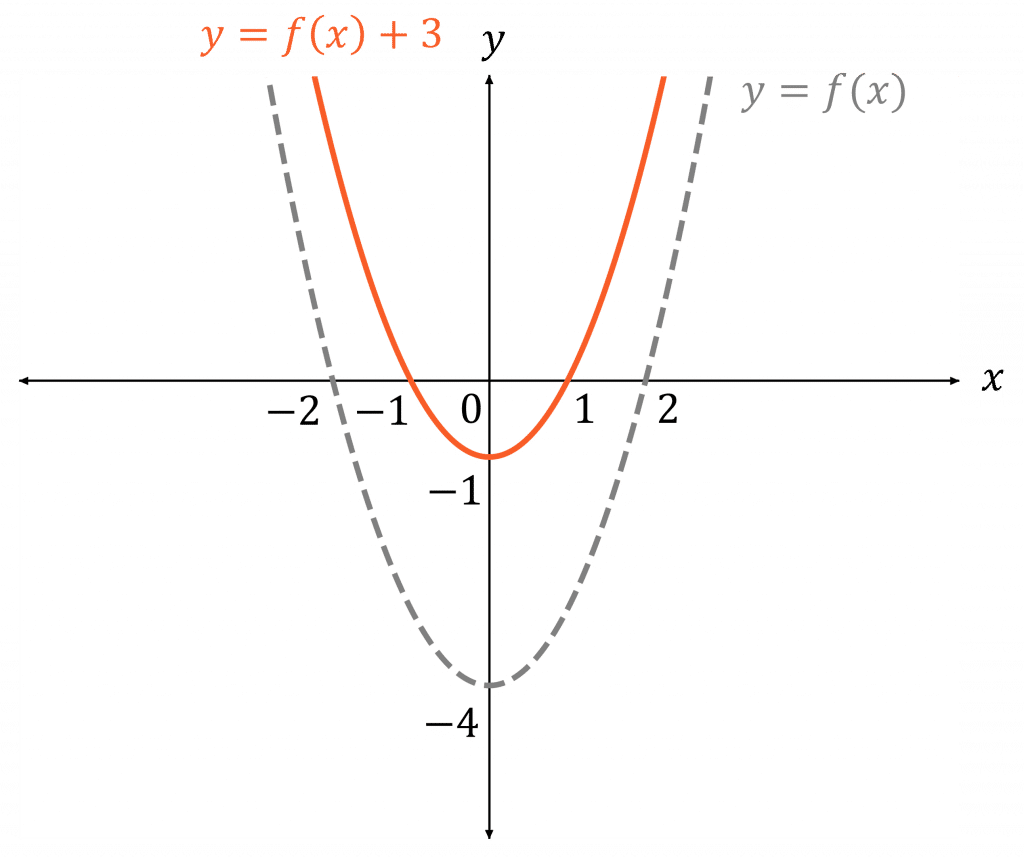For the transformation $y=f(x)+k$, for $k>0$:

• $f(x)+k$ is $f(x)$ moved $k$ upwards
• $f(x)-k$ is $f(x)$ moved $k$ downwards

In this example, we have $f(x) = x^2 - 4$ and $y=f(x)+3$

So, add $3$ to the $y$-coordinates of $f(x)$ to get $y=f(x)+3$

A LevelAQAEdexcelOCR

## Type 3: $y = af(x)$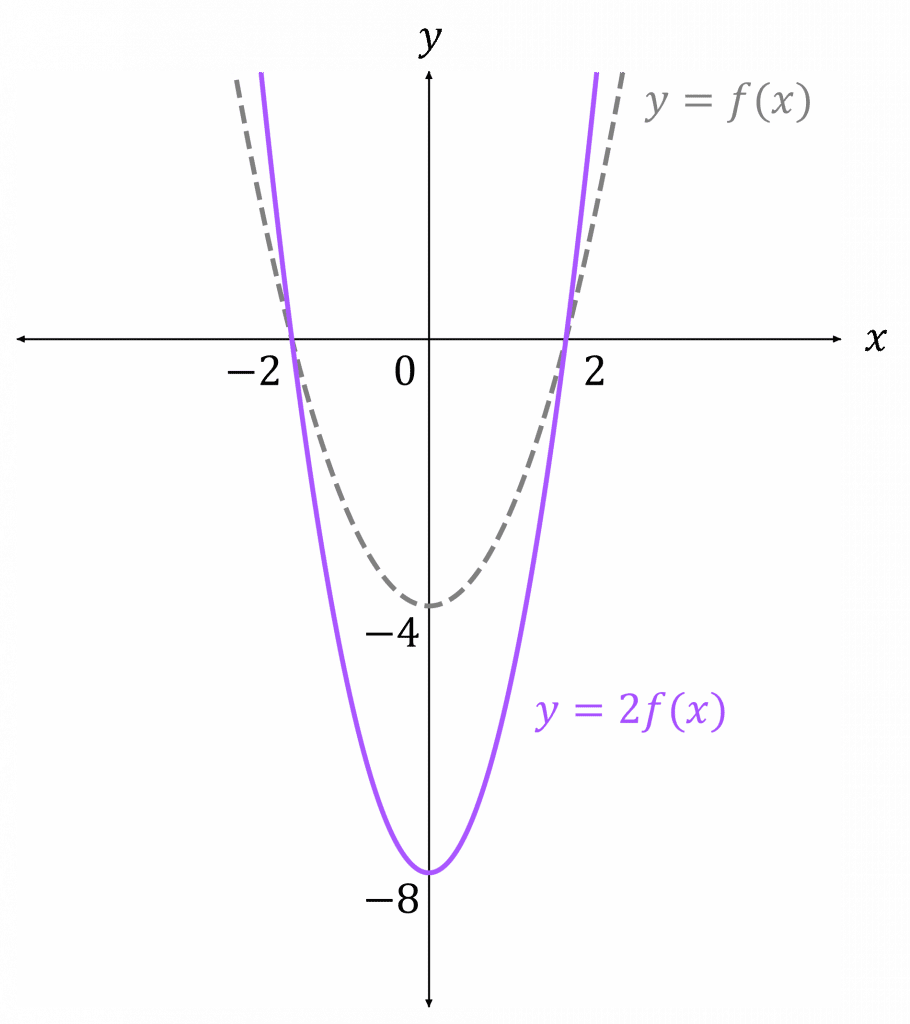For the transformation $y=af(x)$:

• If $|a|>1$ then $af(x)$ is $f(x)$ stretched vertically by a factor of $a$
• If $0<|a|<1$ then $f(x)$ is squashed vertically
• If $a<0$ then is $f(x)$ also reflected in the $x$-axis

In this example, we have $f(x) = x^2 - 4$ and $y=2f(x)$

This is a stretch vertically, so multiply the $y$-coordinates of $f(x)$ by $2$ to get $y=2f(x)$

A LevelAQAEdexcelOCR

## Type 4: $y = f(ax)$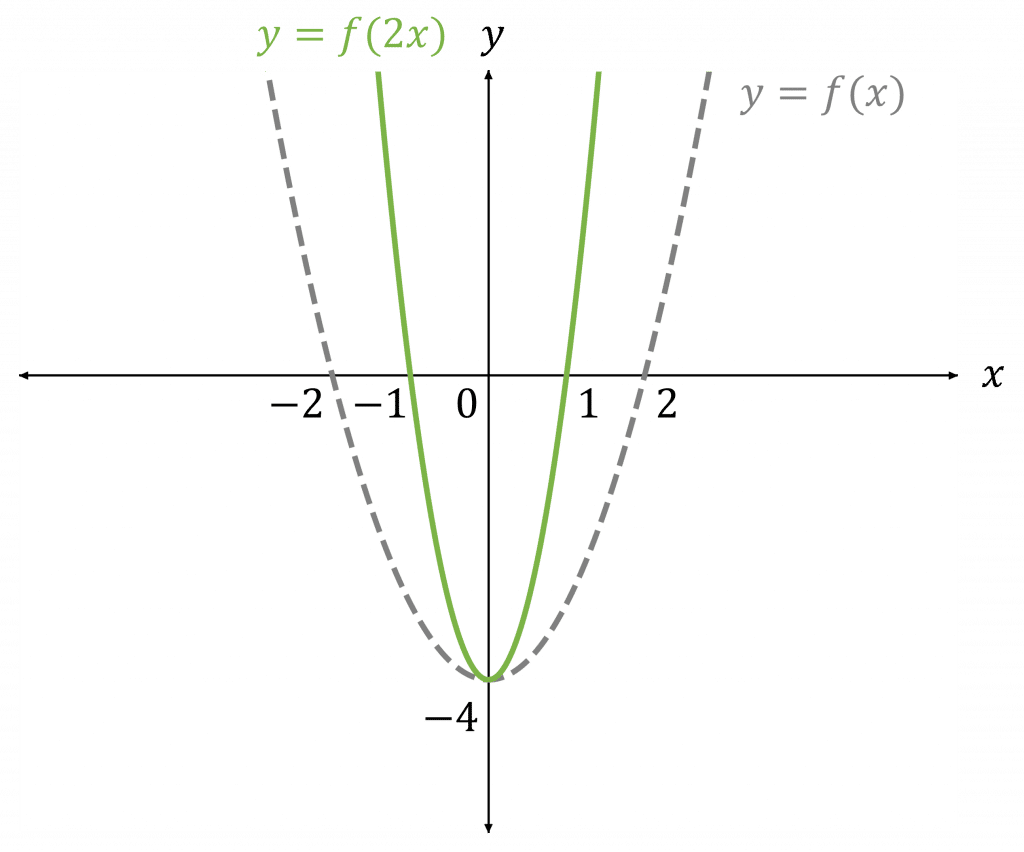For the transformation $y=f(ax)$:

• If $|a|>1$ then $f(ax)$ is $f(x)$ squashed horizontally by a factor of $a$
• If $0<|a|<1$ then $f(x)$ is stretched horizontally
• If $a<0$ then is $f(x)$ also reflected in the $y$-axis

In this example, we have $f(x) = x^2 - 4$ and $y=f(2x)$

This is a squash horizontally, so divide the $x$-coordinates of $f(x)$ by $2$ (or multiply by $\dfrac{1}{2}$) to get $y=f(2x)$

A LevelAQAEdexcelOCR

## Note:

• For these transformations, any asymptotes need to be moved correspondingly.
• A squash by a factor of $a$ is equivalent to a stretch by a factor of $\dfrac{1}{a}$
• When drawing graph transformation, only a sketch including important points is necessary.

## Combinations of Transformations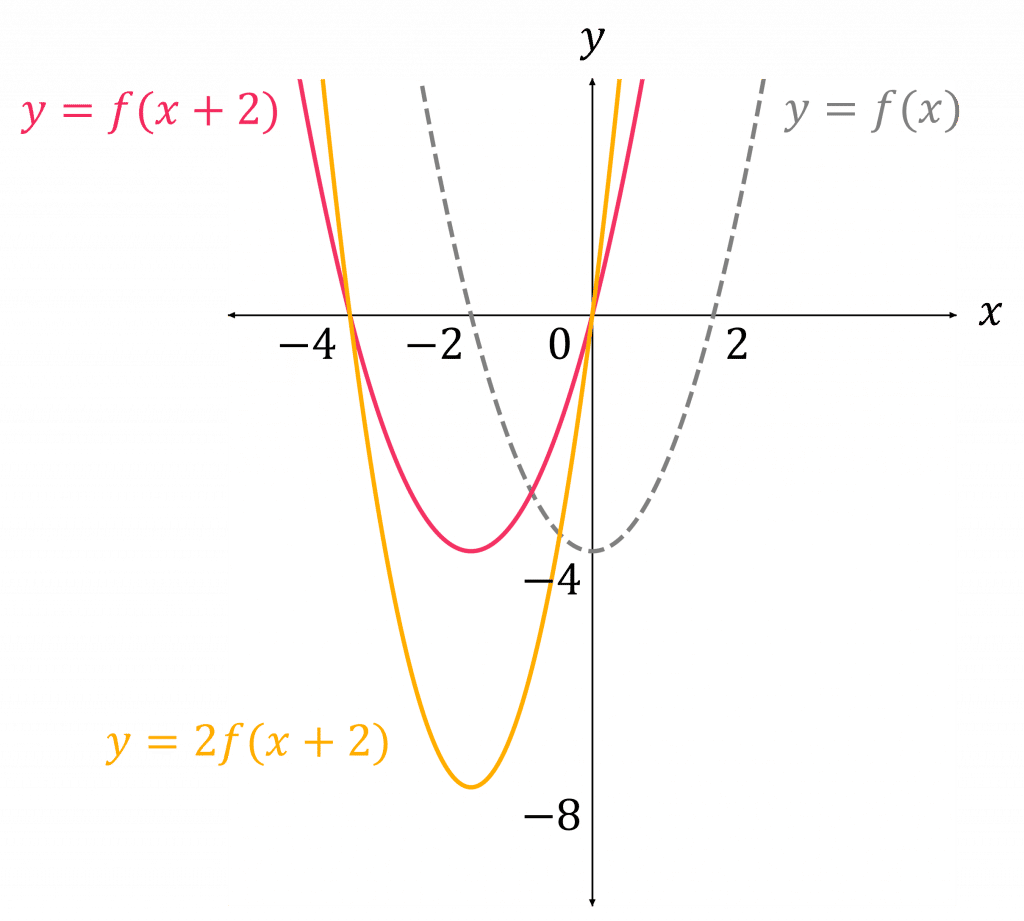For combinations of transformations, it is easy to break them up and do them one step at a time (do the bit in the brackets first). You can sketch the graph at each step to help you visualise the whole transformation.

e.g. for $f(x) = x^2 - 4$ and $y=2f(x+2)$, draw the graph of
$y=f(x+2)$ first, and then use this graph to draw the graph of
$y=2f(x+2)$

Note: These transformations can also be combined with modulus functions.

A LevelAQAEdexcelOCR

## Graph Transformations Example Questions

$y = f(4x)$ means that the graph of $f(x)$ is squashed horizontally by a factor of $4$.

Hence, the graph will look like: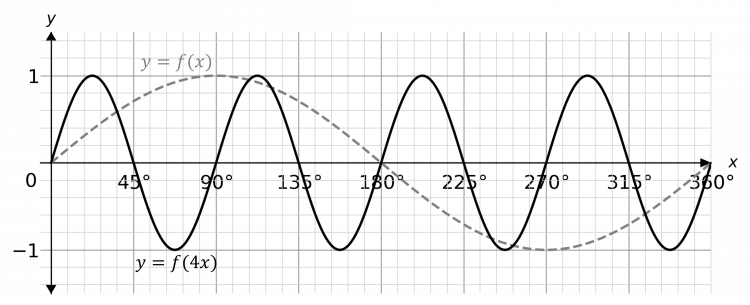Firstly, since the coefficient before $f(x)$ is negative, we need to reflect $f(x)$ in the $x$-axis.

The coefficient of $\dfrac{1}{2}$ before $-f(x)$ means that the graph of $-f(x)$ is squashed vertically by a factor of $2$.

Hence, the graph will look like: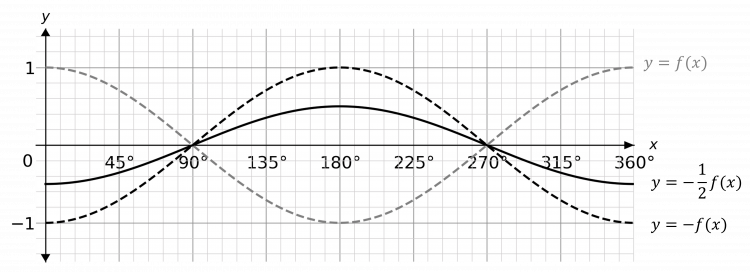Split the transformation up into $2$ parts – firstly sketch $y=3f(x)$ which is a stretch vertically by a scale factor of $3$ (multiply the $y$-coordinates by $3$: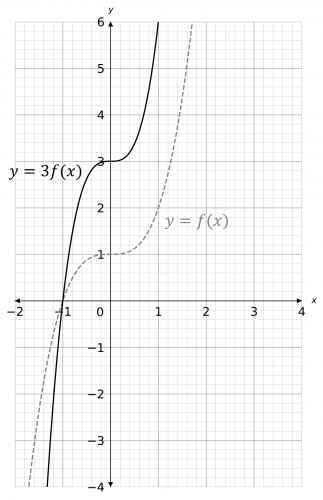Then, do the second transformation – $y=3f(x)-1$ means that we need to move the graph down by $1$ (subtract $1$ from the $y$-coordinates):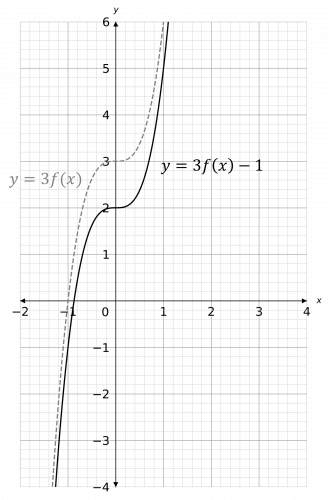A Level

A Level

## You May Also Like...### MME Learning Portal

Online exams, practice questions and revision videos for every GCSE level 9-1 topic! No fees, no trial period, just totally free access to the UK’s best GCSE maths revision platform.

£0.00

A Level

A Level